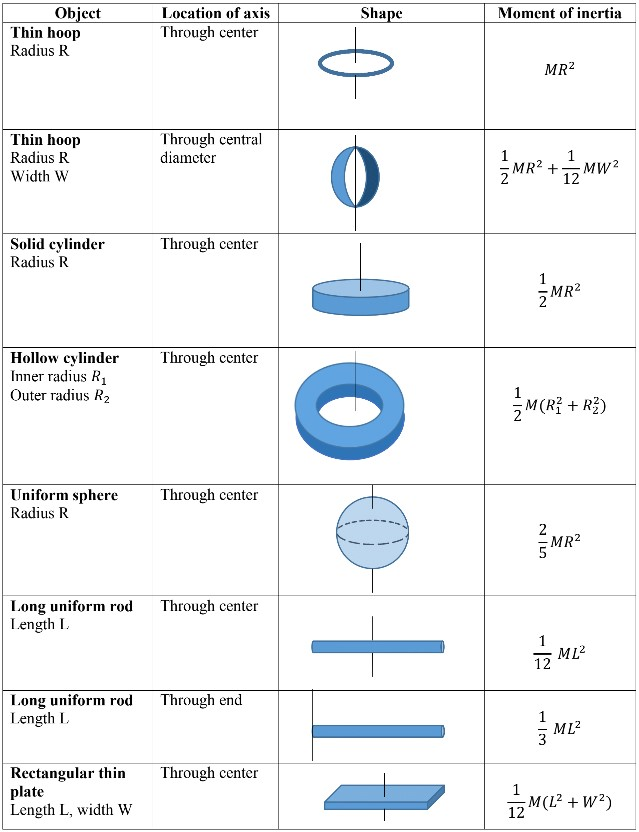# Torque and rotational inertia#### Everything You Need in One Place

Homework problems? Exam preparation? Trying to grasp a concept or just brushing up the basics? Our extensive help & practice library have got you covered.#### Learn and Practice With Ease

Our proven video lessons ease you through problems quickly, and you get tonnes of friendly practice on questions that trip students up on tests and finals.#### Instant and Unlimited Help

Our personalized learning platform enables you to instantly find the exact walkthrough to your specific type of question. Activate unlimited help now!

##### Intros
###### Lessons
1. Definition of "Torque"
2. Rotational Inertia
##### Examples
###### Lessons
1. A person exerts a force of 45N on the handle of a door 65cm wide.
1. What is the magnitude of the torque if the force is exerted perpendicular to the door?
2. What is the magnitude of the torque if the force is exerted at a 35°?
2. Calculate the net torque about the axle of the of the wheel shown below. Assume that a friction torque of 0.60 $N.m$ opposes the motion.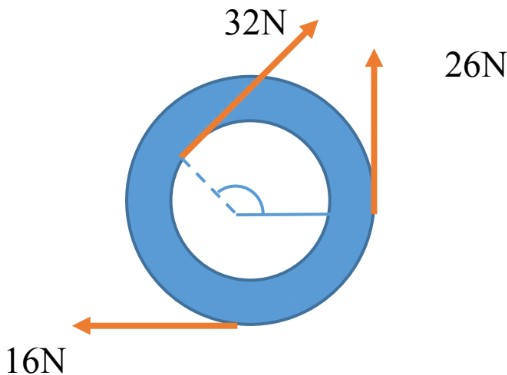1. A torque of 66 $N.m$ is required to tighten the bolts on the cylinder head of a car engine. The wrench used to tighten the bolt is 26cm long.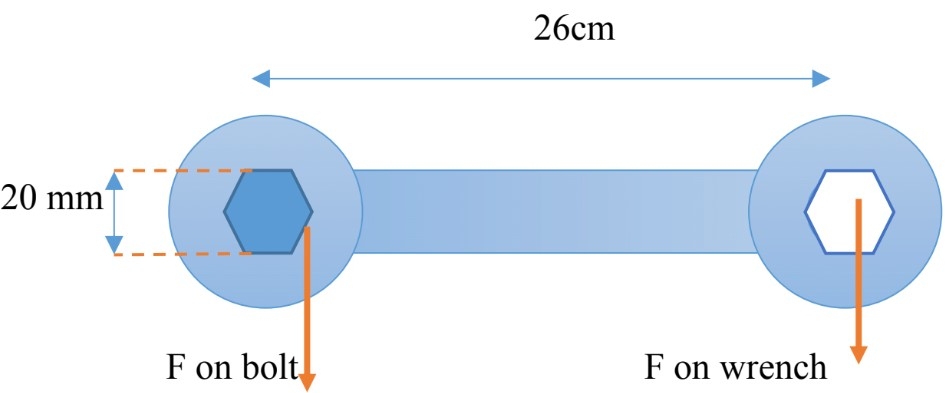1. What is the perpendicular force that is exerted by the mechanics to the end of the wrench to tighten the bolts?
2. If the six-sided bolt head has a diameter of 20mm, how much force will be applied near each of the six points?
2. Two small weights of mass 4.0kg and 6.0kg are mounted 6.0m apart on a light rod as shown below. Calculate the moment of inertia of the system;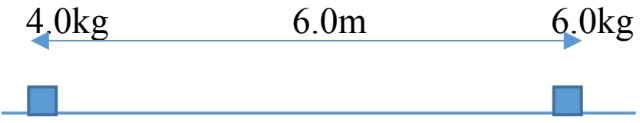1. When rotated about an axis halfway between the weights.
2. When rotated about an axis 0.40m to the left of the 4.0kg mass.
3. A 12.0N force is applied to a cord wrapped around a pulley of mass $M$ = 6.00kg and radius $R$= 22.0cm. The pulley accelerates uniformly from rest to an angular speed of 40.0 rad/s in 2.00s. If there is a frictional torques $\tau _{fr}$ = 1.20$m.N$ at the axle, determine the moment of inertia of the pulley as it rotates around its center.
1. Determine the moment of inertia for the following uniform objects:
1. A 12.80 kg sphere of radius 0.464m rotating through its center.
2. A bicycle wheel 88.2cm in diameter. The rim and tire have a combined mass of 1.36kg
3. A helicopter rotor blade (long thin rod), 2.76 m long and has a mass of 140kg.
2. An Atwood's machine consists of two masses, $m_{1}$ = 4.0kg and $m_{2}$ = 7.0kg, which are connected by a massless inelastic cord that passes over a pulley. The pulley has radius $R$ = 0.4m and moment of inertia $I$ =4.0 kg. $m_{2}$ about its axle.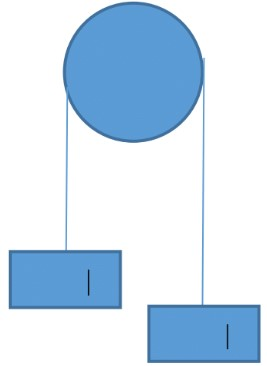1. Determine the acceleration of the masses $m_{1}$ and $m_{2}$.
2. Find tensions supporting $m_{1}$ and $m_{2}$.
###### Free to Join!
StudyPug is a learning help platform covering math and science from grade 4 all the way to second year university. Our video tutorials, unlimited practice problems, and step-by-step explanations provide you or your child with all the help you need to master concepts. On top of that, it's fun - with achievements, customizable avatars, and awards to keep you motivated.
• #### Easily See Your ProgressWe track the progress you've made on a topic so you know what you've done. From the course view you can easily see what topics have what and the progress you've made on them. Fill the rings to completely master that section or mouse over the icon to see more details.
• #### Make Use of Our Learning Aids###### Practice Accuracy

Get quick access to the topic you're currently learning.

See how well your practice sessions are going over time.

Stay on track with our daily recommendations.

• #### Earn Achievements as You LearnMake the most of your time as you use StudyPug to help you achieve your goals. Earn fun little badges the more you watch, practice, and use our service.
• #### Create and Customize Your AvatarPlay with our fun little avatar builder to create and customize your own avatar on StudyPug. Choose your face, eye colour, hair colour and style, and background. Unlock more options the more you use StudyPug.
###### Topic Notes

In this lesson, we will learn:

• Definition of Torque
• Translational equilibrium Vs. Rotational equilibrium
• Rotational Inertia
• Moment of inertia of uniform objects

Notes:

Torque

• To have better understanding of the meaning of “torque”, let’s try the following activity and compare the motion of object as different forces are exerted.
• A ruler is placed on a horizontal flat surface.

• (a) The force is applied at the center of mass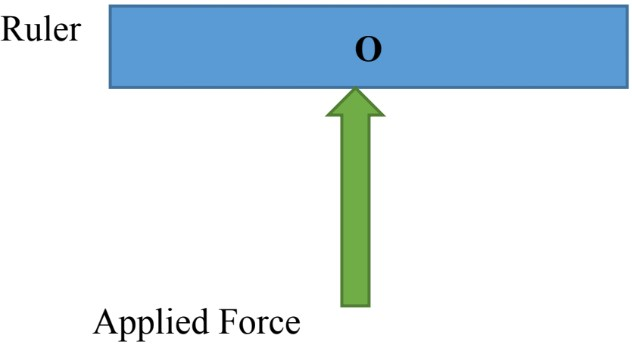$\quad$The whole object (ruler) will accelerate in the direction of the force exerted.

(b) The force is applied away from the center of mass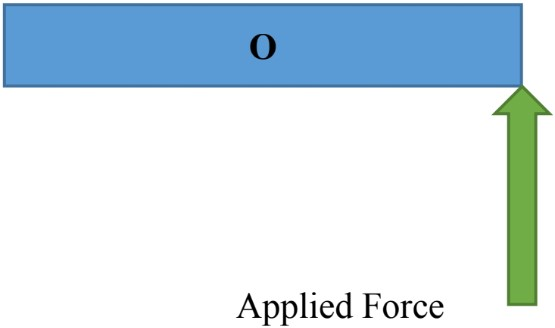The whole object (ruler) will rotate about the center of mass.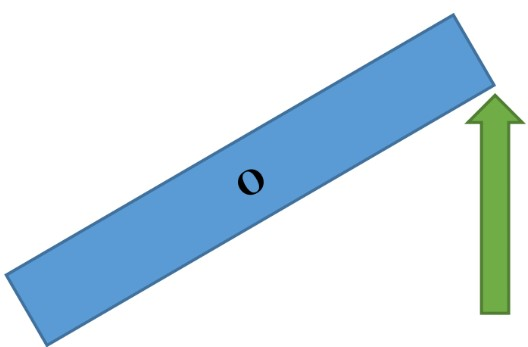As the result of exerting force, the object will rotate about the “Pivot” or “Axis of Rotation”.

Therefore, we can define Torque as the “Turning Effect of a Force”.

Torque is represented by the Greek letter $\tau$ and the standard unit is $N.m.$

Conclusion:
• Force causes acceleration; $F=ma$

• Torque causes angular acceleration: $\tau = r F \sin \theta \;$ (vector quantity)
$\theta$ : angle between $r$ and $F$
$r$: distance between the pivot and point at which the force is exerted on.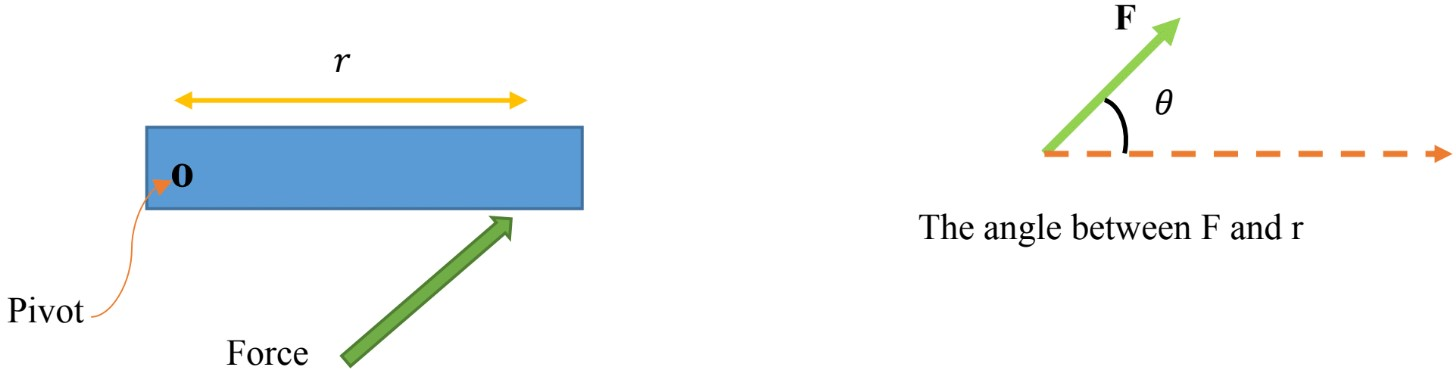$\tau \, \propto \, r$: the further the force, the bigger the torque
$\tau \, \propto \, \theta$: the larger the angle, the bigger the torque

The object could be in Translational Equilibrium and Rotational Equilibrium;

$\sum F = 0 \qquad \qquad$ “Translational Equilibrium” $\; \Rightarrow \,$ acceleration is zero
$\sum \tau = 0 \qquad \qquad$ “Rotational Equilibrium” $\; \Rightarrow \,$ angular acceleration is zero

Rotational Inertia

Let’s consider a mass m rotating in a circle of radius r about a fixed point. The object is going to experience tangential and angular acceleration.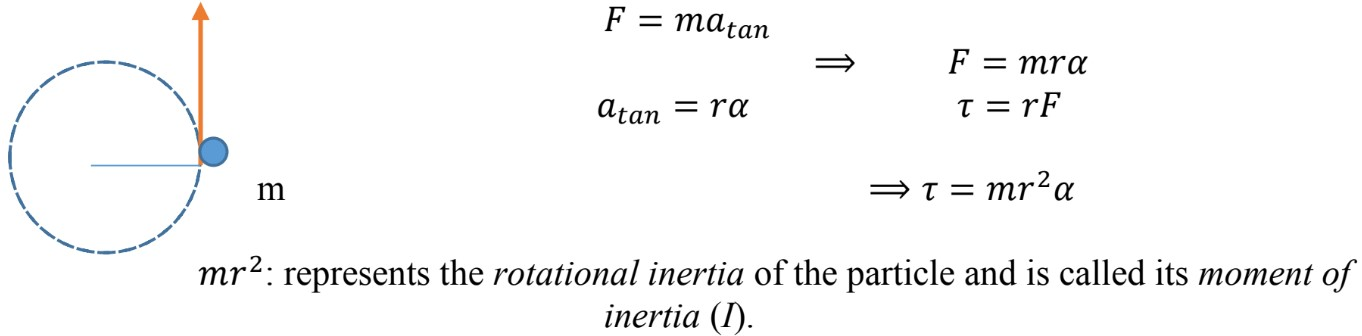$I = mr^{2} \quad$ (scalar quantity) Unit: kg.m2

“Rotational Inertia represents the resistance to angular acceleration”

• Large Rotational Inertia: the mass of the object is distributed far from the axis of rotation.
Objects with larger Rotational Inertia are harder to get rotating and harder to stop rotating.
• Small Rotational Inertia: the mass of the object is distributed close to the axis of rotation.

$I = mr^{2} \qquad$ (single mass rotating of a single radius)
$I = \sum mr^{2} \enspace \;$ (multiple individual masses rotating in circles of different radius)

• Moment of inertia for objects of uniform composition is constant;